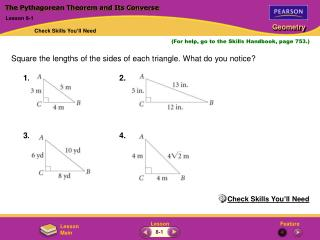# The Pythagorean Theorem and Its Converse - PowerPoint PPT PresentationDownload PresentationThe Pythagorean Theorem and Its Converse

The Pythagorean Theorem and Its ConverseDownload Presentation## The Pythagorean Theorem and Its Converse

- - - - - - - - - - - - - - - - - - - - - - - - - - - E N D - - - - - - - - - - - - - - - - - - - - - - - - - - -
##### Presentation Transcript

1. 1. 2. 3. 4. The Pythagorean Theorem and Its Converse Lesson 8-1 Check Skills You’ll Need (For help, go to the Skills Handbook, page 753.) Square the lengths of the sides of each triangle. What do you notice? Check Skills You’ll Need 8-1

2. 1. 32 = (3)(3) = 9; 42 = (4)(4) = 16; 52 = (5)(5) = 25; 9 + 16 = 25 2. 52 = (5)(5) = 25; 122 = (12)(12) = 144; 132 = (13)(13) = 169; 25 + 144 = 169 3. 62 = (6)(6) = 36; 82 = (8)(8) = 64; 102 = (10)(10) = 100; 36 + 64 = 100 4. 42 = (4)(4) = 16; (4 2)2 = (4 2)(4 2) = 16 (2)(2) = 16(2) = 32; 16 + 16 = 32. The Pythagorean Theorem and Its Converse Lesson 8-1 Check Skills You’ll Need Solutions 8-1

3. The Pythagorean Theorem and Its Converse Lesson 8-1 Notes 8-1

4. The Pythagorean Theorem and Its Converse Lesson 8-1 Notes A Pythagorean triple is a set of nonzero whole numbers a, b, and cthat satisfy the equation a2 + b2 = c2. Some common Pythagorean triples are: 3, 4, 5 5, 12, 13 8, 15, 17 7, 24, 25 8-1

5. The Pythagorean Theorem and Its Converse Lesson 8-1 Notes 8-1

6. The Pythagorean Theorem and Its Converse Lesson 8-1 Notes 8-1

7. The Pythagorean Theorem and Its Converse Lesson 8-1 Notes 8-1

8. A right triangle has legs of length 16 and 30. Find the length of the hypotenuse. Do the lengths of the sides form a Pythagorean triple? The Pythagorean Theorem and Its Converse Lesson 8-1 Additional Examples Pythagorean Triples a2 + b2 = c2Use the Pythagorean Theorem. 162+ 302 = c2Substitute 16 for a and 30 for b. 256 + 900 = c2Simplify. 1156 = c2 34 = cTake the square root. The length of the hypotenuse is 34. The lengths of the sides, 16, 30, and 34, form a Pythagorean triple because they are whole numbers that satisfy a2 + b2 = c2. Notice that each length is twice the common Pythagorean triple of 8, 15, and 17. Quick Check 8-1

9. Find the value of x. Leave your answer in simplest radical form. x = 4(11) Take the square root of each side. x = 2 11 Simplify. The Pythagorean Theorem and Its Converse Lesson 8-1 Additional Examples Using Simplest Radical Form a2 + b2 = c2Use the Pythagorean Theorem. x2 + 102 = 122Substitute x for a, 10 for b, and 12 for c. x2 + 100 = 144 Simplify. x2 = 44 Subtract 100 from each side. Quick Check 8-1

10. A baseball diamond is a square with 90-ft sides. Home plate and second base are at opposite vertices of the square. About how far is home plate from second base? c = 16,200 Take the square root. c 127.27922 Use a calculator. The Pythagorean Theorem and Its Converse Lesson 8-1 Additional Examples Real-World Connection Use the information to draw a baseball diamond. a2 + b2 = c2Use the Pythagorean Theorem. 902 + 902 = c2Substitute 90 for a and for b. 8,100 + 8,100 = c2Simplify. 16,200 = c2 The distance to home plate from second base is about 127 ft. Quick Check 8-1

11. a2 + b2c2 42+ 62 72Substitute 4 for a, 6 for b, and 7 for c. 16 + 36 49 Simplify. The Pythagorean Theorem and Its Converse Lesson 8-1 Additional Examples Is It a Right Triangle? Is this triangle a right triangle? 52 ≠ 49 Because a2 + b2 ≠ c2, the triangle is not a right triangle. Quick Check 8-1

12. c2a2 + b2Compare c2 with a2+ b2. 252 152 + 202 Substitute the greatest length for c. 625 225 + 400 Simplify. The Pythagorean Theorem and Its Converse Lesson 8-1 Additional Examples Classifying Triangles as Obtuse, Acute, or Right The numbers represent the lengths of the sides of a triangle. Classify each triangle as acute, obtuse, or right. a.15, 20, 25 625 = 625 Because c2 = a2 + b2, the triangle is a right triangle. 8-1

13. c2a2 + b2Compare c2 with a2 + b2. 202 102 + 152Substitute the greatest length for c. 400 100 + 225 Simplify. 400 325 Because c2a2 + b2, the triangle is obtuse. The Pythagorean Theorem and Its Converse Lesson 8-1 Additional Examples (Continued) b. 10, 15, 20 Quick Check 8-1

14. 1. Find the value of x. 2. Find the value of x. Leave your answer in simplest radical form. 2 33 The Pythagorean Theorem and Its Converse Lesson 8-1 Lesson Quiz 3. The town of Elena is 24 mi north and 8 mi west of Holberg. A train runs on a straight track between the two towns. How many miles does it cover? Round your answer to the nearest whole number. 4. The lengths of the sides of a triangle are 5 cm, 8 cm, and 10 cm. Is it acute, right, or obtuse? 15 25 mi obtuse 8-1